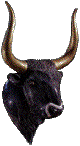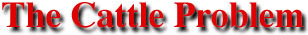S O L U T I O N . ( 2 N D . P A R T )
 Back to . . .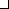Archimedes Home Page This section . . .Statement (English)Solution (1st part)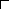Solution (2nd part)Statement (Greek)Computer Output (1st part)Computer Output (2nd part)

The Second Part of the Cattle Problem

The first part of the cattle problem has infinitely many solutions given by

 W = 10,366,482k B = 7,460,514k Y = 4,149,387k D = 7,358,060k w = 7,206,360k b = 4,893,246k y = 5,439,213k d = 3,515,820k

where k can be any positive integer. The second part of the cattle problem imposes two additional conditions that restrict the possible values of k beyond k = 1, 2, 3, ... . The first additional condition states

When the while bulls mingled their number with the black, they stood firm, equal in depth and breadth ...
The most direct interpretation of this condition is that
W + B = a square number
or
10,366,482k + 7,460,514k = a square number
or
17,826,996k = a square number
or
(2)(2)(3)(11)(29)(4657)k = a square number
where, in the last equation, the number 17,826,996 has been expressed as a product of prime numbers. For the left-hand side of this equation to be a square number, it follows that k must be of the form
k = (3)(11)(29)(4657)r2
or
k = 4,456,749r2
where r is any positive integer.

The second additional condition, which further restricts the allowable value of k, states

... when the yellow and the dappled bulls were gathered into one herd they stood in such a manner that their number, beginning from one, grew slowly greater till it completed a triangular figure ...
This means that
Y + D = a triangular number
where triangular numbers are numbers of the form
1 + 2 + 3 + 4 + 5 + . . . + m
where m is some positive integer. By using the formula for the sum of the first m integers, we can also characterize triangular numbers as those numbers of the form
m(m + 1)/2
where m is some positive integer. At this point we have
4,149,387k + 7,358,060k = m(m + 1)/2
or
11,507,447k = m(m + 1)/2
Using our previous condition for the allowable values of k, this becomes
(11,507,447)(4,456,749)r2 = m(m + 1)/2
or
102,571,605,819,606r2 = m(m + 1)
The problem now is to find positive integers r and m that satisfy this last equation. A partial solution to this problem was given in
A. Amthor
"Das Problema bovinum des Archimedes"
Zeitschrift fur Math. u. Physik. (Hist.-litt.Abtheilung)
Volume XXV (1880), pages 153-171
Amthor found that the smallest integers r and m that satisfy this equation result in a total number of cattle expressed by an integer with 206,545 digits that begins with the digits 776.

 “Since it has been calculated that it would take the work of a thousand men for a thousand years to determine the complete number [of cattle], it is obvious that the world will never have a complete solution.” Pre-computer-age thinking from a letter to The New York Times January 18, 1931 Amthor's calculations were continued by an ad hoc group called the Hillsboro Mathematical Club (Hillsboro, Illinois, USA) in the years 1889 to 1893. The club's three members (Edmund Fish, Geo. H. Richards, and A. H. Bell) computed the first 31 digits and the last 12 digits of the smallest total number of cattle and found them to be 7760271406486818269530232833209 . . . 719455081800 However, the two underlined digits should be 13. Their results were published in A. H. Bell “The “Cattle Problem.” ByArchimedies[sic] 251 B.C.” American Mathematical Monthly Volume 2 (1895) pages 140-141IBM 7040 circa 1965 Further progress on the solution had to await the computer age. In 1965 three researchers at the University of Waterloo (Waterloo, Ontario, Canada) announced a complete solution to the cattle problem in H. C. Williams, R. A. German, and C. R. Zarnke “Solution of the cattle problem of Archimedes” Mathematics of Computation Volume XIX (1965) pages 671-674 Their calculations required 7 hours and 49 minutes of computing time, mainly on an IBM 7040, and their results were deposited in the Unpublished Mathematical Tables file of the above journal.Hugh Williams, Gus German, and Robert Zarnke 1965Cray 1 circa 1976 In 1981 Harry L. Nelson of the Lawrence Livermore National Laboratory (Livermore, California, USA) published the 47-page printout from a CRAY 1 computer containing the 206,545 digits of the smallest possible value for the total number of cattle in Harry L. Nelson “A solution to Archimedes' cattle problem” Journal of Recreational Mathematics Volume 13 (1980-81) pages 162-176 Nelson's computations were performed as part of the testing and validation of the laboratory's newly delivered Cray 1. The computations, together with extensive checking, took about ten minutes. In addition to the smallest solution, five additional solutions were found to further test the computer, the largest containing over a million digits.

In 1998 Ilan Vardi of Occidental College (Los Angeles, California, USA) developed simple explicit formulas to generate solutions to the cattle problem. His results appear in

Ilan Vardi
“Archimedes’ cattle problem”
American Mathematical Monthly
Volume 105 (April 1998) pages 305-319

(Download a PDF file of a preprint of this paper : 148 kilobytes)
(Download a postscript file of a preprint of this paper : 695 kilobytes)

In particular, he derived the result that the smallest possible value for the total number of cattle can be written aswherexdenotes the smallest integer greater than or equal to x. You can also read Ivars Peterson's synopsis of this paper in his MathTrek article of April 18, 1998.

Another approach to this problem can be found in

Antti Nygrén
“A simple solution to Archimedes’ cattle problem”
University of Oulu
Linnanmaa, Oulu, Finland
Acta Universitatis Ouluensis
Scientiae Rerum Naturalium
ISBN 951-42-5932-7, March 2001

Nygrén's algorithm computes the smallest total number of cattle, T, through the following pair of formulas: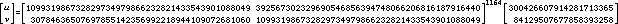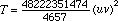These formulas involve only integer arithmetic and can be evaluated on a personal computer in seconds (e.g., five seconds on a Pentium II notebook computer running Maple or Mathematica).

The first and last fifty digits of the smallest total number of cattle found by these researchers are

77602714064868182695302328332138866642323224059233
. . .

05994630144292500354883118973723406626719455081800

There are few numerical problems in mathematics that have taken 22 centuries to solve.